# The CALIS Procedure

### Example 29.17 Path Analysis: Stability of Alienation

The following covariance matrix from Wheaton et al. (1977) has served to illustrate the performance of several implementations for the analysis of structural equation models. Two different models have been analyzed by an early implementation of LISREL and are mentioned in Jöreskog (1978). You can also find a more detailed discussion of these models in the LISREL VI manual (Jöreskog and Sörbom 1985). A slightly modified model for this covariance matrix is included in the EQS 2.0 manual (Bentler 1985, p. 28). However, for the analysis with the EQS implementation, the SEI variable is rescaled by a factor of 0.1 to make the matrix less ill-conditioned. Since the Levenberg-Marquardt or Newton-Raphson optimization techniques are used with PROC CALIS, rescaling the data matrix is not necessary and, therefore, is not done here. The results reported here reflect the estimates based on the original covariance matrix.

The path diagram of this model is displayed in Figure 29.1 and is reproduced in the following:

Output 29.17.1: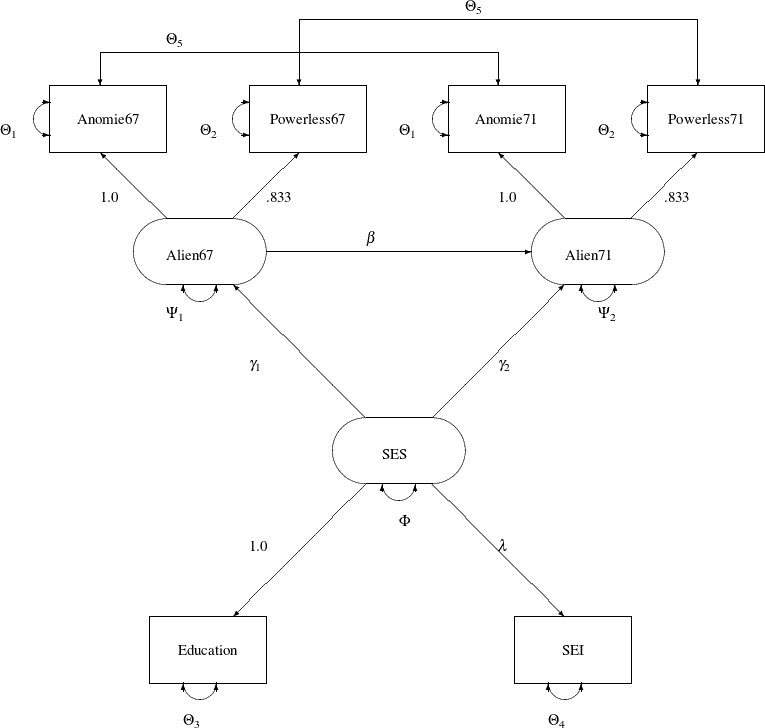You use the PATH modeling language of PROC CALIS to specify this path model, as shown in the following statements:

title "Stability of Alienation";
title2 "Data Matrix of WHEATON, MUTHEN, ALWIN & SUMMERS (1977)";
data Wheaton(TYPE=COV);
_type_ = 'cov';
input _name_ \$ 1-11 Anomie67 Powerless67 Anomie71 Powerless71
Education SEI;
label Anomie67='Anomie (1967)' Powerless67='Powerlessness (1967)'
Anomie71='Anomie (1971)' Powerless71='Powerlessness (1971)'
Education='Education'    SEI='Occupational Status Index';
datalines;
Anomie67       11.834     .        .        .       .        .
Powerless67     6.947    9.364     .        .       .        .
Anomie71        6.819    5.091   12.532     .       .        .
Powerless71     4.783    5.028    7.495    9.986    .        .
Education      -3.839   -3.889   -3.841   -3.625   9.610     .
SEI           -21.899  -18.831  -21.748  -18.775  35.522  450.288
;

ods graphics on;

proc calis nobs=932 data=Wheaton plots=residuals;
path
Anomie67   Powerless67  <===  Alien67   = 1.0  0.833,
Anomie71   Powerless71  <===  Alien71   = 1.0  0.833,
Education  SEI          <===  SES       = 1.0  lambda,
Alien67    Alien71      <===  SES       = gamma1 gamma2,
Alien71                 <===  Alien67   = beta;
pvar
Anomie67     = theta1,
Powerless67  = theta2,
Anomie71     = theta1,
Powerless71  = theta2,
Education    = theta3,
SEI          = theta4,
Alien67      = psi1,
Alien71      = psi2,
SES          = phi;
pcov
Anomie67    Anomie71    = theta5,
Powerless67 Powerless71 = theta5;
pathdiagram title='Stability of Alienation';
run;

ods graphics off;


Since no METHOD= option is used in the PROC CALIS statement, maximum likelihood estimates are computed by default.

In the PATH statement, you specify the functional relationships of the variables in the model. These functional relationships are represented as single-headed paths in the path diagram. There are five entries in the PATH statement. You specify the relationships between the latent constructs and the observed variables in the first three path entries. For example, the first entry states that Anomie and Powerless67 are measured indicators of the latent variable Alien67. The path effects or coefficients from the latent factor to these measured indicators are fixed at 1.0 and 0.833, respectively. Similarly, in the next two path entries, you define the relationships between the latent factors Alien71 and SES and their measured indicators. The last two path entries in the PATH statement represent the functional relationships among the latent variables in the model. SES has effects on Alien67 and Alien71. These effect parameters are labeled or named with gamma1 and gamma2, respectively. Alien67 also has an effect on Alien71, with the effect parameter named beta.

In the PVAR statement, you specify the variance or error variance parameters in the model. These parameters correspond to the double-headed arrows pointing to the individual variables in the path diagram. In the first six entries of the PVAR statement, you specify the error variance parameters of the observed variables. You also give names to these parameters that correspond to the notation in the path diagram. Although you can choose any names for the parameters, it is important to remember that parameters with the same name are identical and will have the same estimates. For example, the error variances of Anomie67 and Anomie71 are the same parameter named theta1. Similarly, you constrain the error variances of Powerless67 and Powerless71. However, the error variance parameters of Education and SEI are unique. They are not constrained with other parameters in the model because they have unique parameter names. Next, you specify the error variance parameters of Alien67 and Alien71. They also have unique parameter names and therefore they are not constrained with any other parameters in the model. Lastly, you specify the variance parameter phi of SES.

In the PCOV statement, you specify the covariances or error covariances among variables in the model. These parameters correspond to the double-headed arrows pointing to distinct pairs of variables in the path diagram. Observed variables Anomie67 and Anomie71 have correlated errors and you specify this error covariance parameter as theta5. Similarly, observed variables Powerless67 and Powerless71 have correlated errors and you also specify this error covariance parameter as theta5. This way, the two error covariances are constrained to be equal.

PROC CALIS can produce a high-quality residual histogram that is useful for showing the distribution of residuals. Before you request the residual histogram, ODS Graphics must be enabled. For example, you can specify the ODS GRAPHICS ON statement, as shown in the preceding statements before the PROC CALIS statement. Then, the residual histogram is requested by the plots=residuals option in the PROC CALIS statement. PROC CALIS can also produce a high-quality path diagram for the model. You can use the PATHDIAGRAM statement to request the path diagram and to specify related options.

Output 29.17.2 displays the modeling information and variables in the analysis.

Output 29.17.2: Model Specification and Variables

 PATH Model Specification

The CALIS Procedure
Covariance Structure Analysis: Model and Initial Values

Modeling Information
Maximum Likelihood Estimation
Data Set WORK.WHEATON
N Obs 932
Model Type PATH
Analysis Covariances

Variables in the Model
Endogenous Manifest Anomie67 Anomie71 Education Powerless67 Powerless71 SEI
Latent Alien67 Alien71
Exogenous Manifest
Latent SES

Output 29.17.2 shows that the data set Wheaton was used with 932 observations. The model is specified with the PATH modeling language. Variables in the model are classified into different categories according to their roles. All manifest variables are endogenous in the model. Also, three latent variables are hypothesized in the model: Alien67, Alien71, and SES. While Alien67 and Alien71 are endogenous, SES is exogenous in the model.

Output 29.17.3 echoes the initial specification of the PATH model.

Output 29.17.3: Initial Estimates

Initial Estimates for PATH List
Path Parameter Estimate
Anomie67 <=== Alien67   1.00000
Powerless67 <=== Alien67   0.83300
Anomie71 <=== Alien71   1.00000
Powerless71 <=== Alien71   0.83300
Education <=== SES   1.00000
SEI <=== SES lambda .
Alien67 <=== SES gamma1 .
Alien71 <=== SES gamma2 .
Alien71 <=== Alien67 beta .

Initial Estimates for Variance Parameters
Variance
Type
Variable Parameter Estimate
Error Anomie67 theta1 .
Powerless67 theta2 .
Anomie71 theta1 .
Powerless71 theta2 .
Education theta3 .
SEI theta4 .
Alien67 psi1 .
Alien71 psi2 .
Exogenous SES phi .

Initial Estimates for Covariances Among Errors
Error of Error of Parameter Estimate
Anomie67 Anomie71 theta5 .
Powerless67 Powerless71 theta5 .

The numerical values for estimates in Output 29.17.3 are the initial values that you input in the model specification. A blank value for the associated parameter name for a numerical estimate indicates that the estimate is a fixed value, which would not be changed in the estimation. For example, the first five paths have fixed path coefficients, and their fixed values are shown in the Estimate column. For numerical estimates that have specified parameter names, the numerical values serve as initial values, which would be changed during the estimation. Output 29.17.3 does not actually have this type of specification. Missing values '.' are specified as initial values for all the free parameters that are specified in the model. For example, lambda, gamma1, theta1, and psi1, among others, are free parameters that do not have specified initial values. PROC CALIS automatically generates the initial values of these parameters.

You can examine this output to ensure that the desired model is being analyzed. PROC CALIS outputs the initial specifications or the estimation results in the order you specify in the model, unless you use reordering options such as ORDERSPEC and ORDERALL . Therefore, the input order of specifications is important—it determines how your output would look.

Simple descriptive statistics are displayed in Output 29.17.4.

Output 29.17.4: Descriptive Statistics

Simple Statistics
Variable Mean Std Dev
Anomie67 Anomie (1967) 0 3.44006
Powerless67 Powerlessness (1967) 0 3.06007
Anomie71 Anomie (1971) 0 3.54006
Powerless71 Powerlessness (1971) 0 3.16006
Education Education 0 3.10000
SEI Occupational Status Index 0 21.21999

Because the input data set contains only the covariance matrix, the means of the manifest variables are assumed to be zero. Note that this has no impact on the estimation, unless a mean structure model is being analyzed.

Initial estimates are necessary in all kinds of optimization problems. You can provide these initial estimates or let PROC CALIS to generate them automatically. As shown in Output 29.17.3, you did not provide any initial estimates for the parameters. PROC CALIS uses a combination of well-behaved mathematical methods to complete the initial estimation. The initial estimation methods for the current analysis are shown in Output 29.17.5.

Output 29.17.5: Optimization Starting Point

Initial Estimation Methods
1 Instrumental Variables Method
2 McDonald Method
3 Two-Stage Least Squares

Optimization Start
Parameter Estimates
N Parameter Estimate Gradient
1 lambda 4.99508 -0.00206
2 gamma1 -0.62322 -0.04069
3 gamma2 -0.20437 -0.03816
4 beta 0.66589 0.03789
5 theta1 3.51433 -0.00409
6 theta2 3.65991 0.01182
7 theta3 2.49860 -0.00578
8 theta4 272.85274 0.0000194
9 psi1 5.57764 -0.00217
10 psi2 3.79636 -0.00935
11 phi 7.11140 0.00108
12 theta5 0.45298 -0.06463

In this example, the instrumental variable Method, the McDonald and Hartmann method, and the two-stage least squares method have been used for initial estimation. In the same output, the vector of initial parameter estimates and their gradients are also shown. The initial objective function value is 0.0366.

Output 29.17.6 displays the optimization information, including technical details, iteration history and convergence status.

Output 29.17.6: Optimization

Optimization Start
Active Constraints 0 Objective Function 0.0365979443
Max Abs Gradient Element 0.0646338767 Radius 1

Iteration   Restarts Function
Calls
Active
Constraints
Objective
Function
Objective
Function
Change
Max Abs
Element
Lambda Ratio
Between
Actual
and
Predicted
Change
1   0 4 0   0.01453 0.0221 0.00142 0 1.013
2   0 6 0   0.01448 0.000046 0.000249 0 1.001
3   0 8 0   0.01448 1.007E-7 4.717E-6 0 1.006

Optimization Results
Iterations 3 Function Calls 11
Jacobian Calls 5 Active Constraints 0
Objective Function 0.0144844814 Max Abs Gradient Element 4.7172823E-6
Lambda 0 Actual Over Pred Change 1.0060912391

 Convergence criterion (ABSGCONV=0.00001) satisfied.

The convergence status is important for the validity of your solution. In most cases, you should interpret your results only when the solution is converged. In this example, you obtain a converged solution, as shown in the message at the bottom of the table. The final objective function value is 0.01448, which is the minimized function value during the optimization. If problematic solutions such as nonconvergence are encountered, PROC CALIS issues an error message.

The fit summary statistics are displayed in Output 29.17.7. By default, PROC CALIS displays all available fit indices and modeling information.

Output 29.17.7: Fit Summary

Fit Summary
Modeling Info Number of Observations 932
Number of Variables 6
Number of Moments 21
Number of Parameters 12
Number of Active Constraints 0
Baseline Model Function Value 2.2894
Baseline Model Chi-Square 2131.4327
Baseline Model Chi-Square DF 15
Pr > Baseline Model Chi-Square <.0001
Absolute Index Fit Function 0.0145
Chi-Square 13.4851
Chi-Square DF 9
Pr > Chi-Square 0.1419
Z-Test of Wilson & Hilferty 1.0754
Hoelter Critical N 1169
Root Mean Square Residual (RMR) 0.2281
Standardized RMR (SRMR) 0.0150
Goodness of Fit Index (GFI) 0.9953
Parsimony Index Adjusted GFI (AGFI) 0.9890
Parsimonious GFI 0.5972
RMSEA Estimate 0.0231
RMSEA Lower 90% Confidence Limit 0.0000
RMSEA Upper 90% Confidence Limit 0.0470
Probability of Close Fit 0.9705
ECVI Estimate 0.0405
ECVI Lower 90% Confidence Limit 0.0357
ECVI Upper 90% Confidence Limit 0.0556
Akaike Information Criterion 37.4851
Bozdogan CAIC 107.5330
Schwarz Bayesian Criterion 95.5330
McDonald Centrality 0.9976
Incremental Index Bentler Comparative Fit Index 0.9979
Bentler-Bonett NFI 0.9937
Bentler-Bonett Non-normed Index 0.9965
Bollen Normed Index Rho1 0.9895
Bollen Non-normed Index Delta2 0.9979
James et al. Parsimonious NFI 0.5962

First, the fit summary table starts with some basic modeling information, as shown in Output 29.17.7. You can check the number of observations, number of variables, number of moments being fitted, number of parameters, number of active constraints in the solution, and the independent model chi-square and its degrees of freedom in this modeling information category. Next, three types of fit indices are shown: absolute, parsimony, and incremental.

The absolute indices are fit measures that you interpret them without referring to any baseline model. These indices do not adjust for model parsimony. They always favor models with a large number of parameters. The chi-square test statistic is the best-known absolute index in this category. In this example, the p-value of the chi-square is 0.1419, which is greater than the conventional 0.05 value. From the statistical hypothesis testing point of view, you cannot reject this model. The Z-test of Wilson and Hilferty is also insignificant at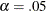, which echoes the result of the chi-square test. You can consult other absolute indices as well. Although it seems that there are no clear conventional levels for these absolute indices to indicate an acceptable model fit, you can always use these indices to compare the relative fit among competing models.

Next, the parsimony fit indices take the model parsimony into account. These indices adjust the model fit by the degrees of freedom (or the number of the parameters) of the model in certain ways. The advantage of these indices is that merely increasing the number of parameters in the model might not necessarily lead better model fit measures. These fit indices penalize models with large numbers of parameters. There is no universal way to interpret all these indices. However, for the relatively well-known RMSEA estimate, by convention values under 0.05 indicate good model fit. The RMSEA value for this example is 0.0231, and so this is a very good model fit. For interpretations of other parsimony indices, you can consult the original articles for these indices.

Last, the incremental fit indices are computed based on comparing the target model fit against the fit of a baseline model, which is usually the so-called uncorrelatedness model where all manifest variables are assumed to be uncorrelated. This is the baseline model that PROC CALIS uses. The baseline model fit statistic is shown under the 'Modeling Info' category of the same fit summary table. In this example, the model fit chi-square of the baseline model is 2131.43, with 15 degrees of freedom. The incremental indices show how well the hypothesized model improves over the baseline model for the data. Various incremental fit indices have been proposed. In the fit summary table, there are six of such fit indices. Large values for these indices are desired. It has been suggested that values greater than .9 for these indices indicate acceptable model fit. In this example, all incremental indices but James et al. parsimonious NFI show that the hypothesized model fits well.

There is no consensus as to which fit index is the best to judge model fit. Probably, with artificial data and model, all fit indices can be shown defective in some aspects of measuring model fit. Conventional wisdom is to look at all fit indices and determine whether the majority of them are close to the desirable ranges of values. In this example, almost all fit indices are good, and so it is safe to conclude that the model fits well.

Nowadays, most researchers pay less attention to the model fit chi-square statistic because it tends to reject all meaningful models with minimum departures from the truth. Although the model fit chi-square test statistic is an impeccable statistical inference tool when the underlying statistical assumptions are satisfied, for practical purposes it is just too powerful to accept any useful and reasonable models with only tiny imperfections. Some fit indices are more popular than others. Standardized RMR, RMSEA estimate, adjusted AGFI, and Bentler’s comparative fit index are frequently reported in empirical research for judging model fit. In this example, all these measures show good model fit of the hypothesized model. While there are certainly legitimate reasons why these fit indices are more popular than others, they are out of the current scope of discussion.

Output 29.17.8 shows the path diagram of the unstandardized solution. The path diagram indicates significant estimates by attaching asterisks to the numerical values. Estimates that are flagged with one asterisk are significant at 0.05-level. Estimates that are flagged with two asterisks are significant at 0.01-level. The path diagram also shows a summary of fit statistics. For more information about specifying path diagram output, see the section Path Diagrams: Layout Algorithms, Default Settings, and Customization.

Output 29.17.8: Path Diagram and Fit Summary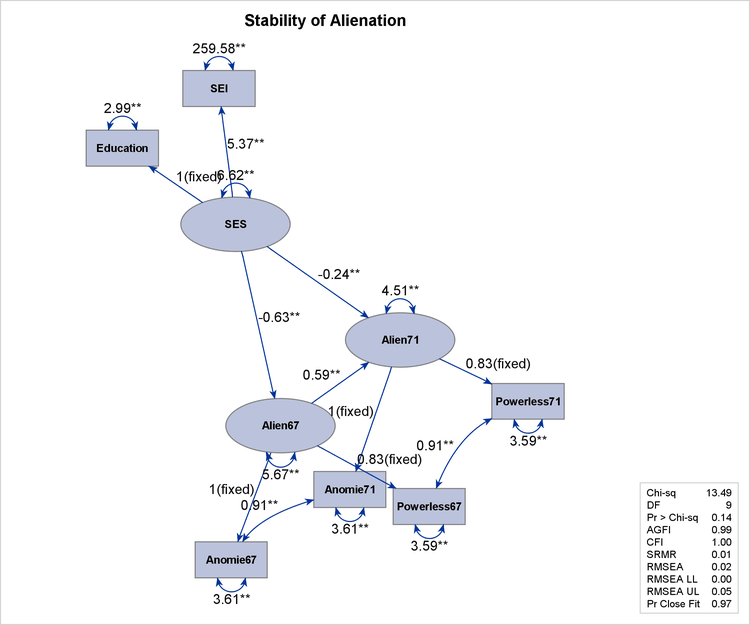PROC CALIS can perform a detailed residual analysis. Large residuals might indicate misspecification of the model. In Output 29.17.9, raw residuals are reported and ranked.

Output 29.17.9: Raw Residuals and Ranking

Raw Residual Matrix
Anomie67 Powerless67 Anomie71 Powerless71 Education SEI
Anomie67 Anomie (1967) -0.06997 0.03642 -0.01116 -0.15200 0.32892 0.47786
Powerless67 Powerlessness (1967) 0.03642 0.01261 0.15600 0.01135 -0.41712 -0.19108
Anomie71 Anomie (1971) -0.01116 0.15600 -0.08381 -0.00854 0.22464 0.07976
Powerless71 Powerlessness (1971) -0.15200 0.01135 -0.00854 0.14067 -0.23832 -0.59248
Education Education 0.32892 -0.41712 0.22464 -0.23832 0.00000 0.00000
SEI Occupational Status Index 0.47786 -0.19108 0.07976 -0.59248 0.00000 0.00002

 Average Absolute Residual 0.15394 0.195044

Rank Order of the 10 Largest Raw Residuals
Var1 Var2 Residual
SEI Powerless71 -0.59248
SEI Anomie67 0.47786
Education Powerless67 -0.41712
Education Anomie67 0.32892
Education Powerless71 -0.23832
Education Anomie71 0.22464
SEI Powerless67 -0.19108
Anomie71 Powerless67 0.15600
Powerless71 Anomie67 -0.15200
Powerless71 Powerless71 0.14067

Because of the differential scaling of the variables, it is usually more useful to examine the standardized residuals instead. In Output 29.17.10, for example, the table for the 10 largest asymptotically standardized residuals is displayed.

Output 29.17.10: Asymptotically Standardized Residuals and Ranking

Asymptotically Standardized Residual Matrix
Anomie67 Powerless67 Anomie71 Powerless71 Education SEI
Anomie67 Anomie (1967) -0.30882 0.52686 -0.05619 -0.86507 2.55338 0.46484
Powerless67 Powerlessness (1967) 0.52686 0.05464 0.87613 0.05735 -2.76371 -0.17015
Anomie71 Anomie (1971) -0.05619 0.87613 -0.35460 -0.12169 1.69781 0.07009
Powerless71 Powerlessness (1971) -0.86507 0.05735 -0.12169 0.58521 -1.55750 -0.49608
Education Education 2.55338 -2.76371 1.69781 -1.55750 0.00000 0.00000
SEI Occupational Status Index 0.46484 -0.17015 0.07009 -0.49608 0.00000 0.00000

 Average Standardized Residual 0.646672 0.818456

Rank Order of the 10 Largest Asymptotically Standardized Residuals
Var1 Var2 Residual
Education Powerless67 -2.76371
Education Anomie67 2.55338
Education Anomie71 1.69781
Education Powerless71 -1.55750
Anomie71 Powerless67 0.87613
Powerless71 Anomie67 -0.86507
Powerless71 Powerless71 0.58521
Powerless67 Anomie67 0.52686
SEI Powerless71 -0.49608
SEI Anomie67 0.46484

The model performs the poorest concerning the covariances of Education with all measures of Powerless and Anomie. This might suggest a misspecification of the functional relationships of Education with other variables in the model. However, because the model fit is quite good, such a possible misspecification should not be a serious concern in the analysis.

The histogram of the asymptotically standardized residuals is displayed in Output 29.17.11, which also shows the normal and kernel approximations.

Output 29.17.11: Distribution of Asymptotically Standardized Residuals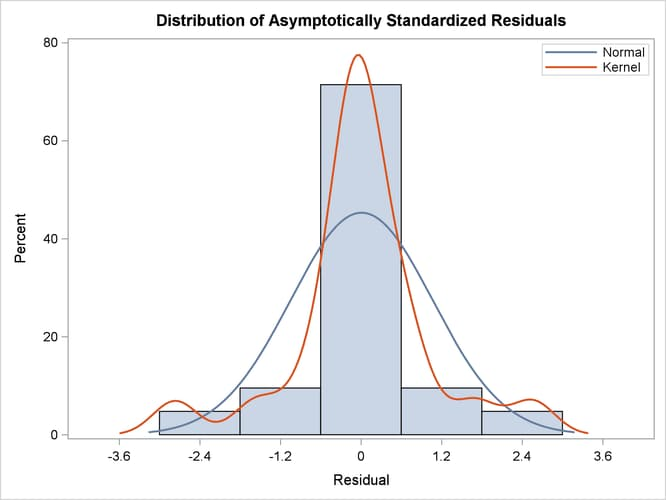The residual distribution looks quite symmetrical. It shows a small to medium departure from the normal distribution, as evidenced by the discrepancies between the kernel and the normal distribution curves.

Output 29.17.12 shows the estimation results.

Output 29.17.12: Estimation Results

PATH List
Path Parameter Estimate Standard
Error
t Value Pr > |t|
Anomie67 <=== Alien67   1.00000
Powerless67 <=== Alien67   0.83300
Anomie71 <=== Alien71   1.00000
Powerless71 <=== Alien71   0.83300
Education <=== SES   1.00000
SEI <=== SES lambda 5.36883 0.43371 12.3788 <.0001
Alien67 <=== SES gamma1 -0.62994 0.05634 -11.1809 <.0001
Alien71 <=== SES gamma2 -0.24086 0.05489 -4.3884 <.0001
Alien71 <=== Alien67 beta 0.59312 0.04678 12.6788 <.0001

Variance Parameters
Variance
Type
Variable Parameter Estimate Standard
Error
t Value Pr > |t|
Error Anomie67 theta1 3.60796 0.20092 17.9572 <.0001
Powerless67 theta2 3.59488 0.16448 21.8556 <.0001
Anomie71 theta1 3.60796 0.20092 17.9572 <.0001
Powerless71 theta2 3.59488 0.16448 21.8556 <.0001
Education theta3 2.99366 0.49861 6.0040 <.0001
SEI theta4 259.57639 18.31151 14.1756 <.0001
Alien67 psi1 5.67046 0.42301 13.4050 <.0001
Alien71 psi2 4.51479 0.33532 13.4639 <.0001
Exogenous SES phi 6.61634 0.63914 10.3519 <.0001

Covariances Among Errors
Error of Error of Parameter Estimate Standard
Error
t Value Pr > |t|
Anomie67 Anomie71 theta5 0.90580 0.12167 7.4447 <.0001
Powerless67 Powerless71 theta5 0.90580 0.12167 7.4447 <.0001

The paths, variances and partial (or error) variances, and covariances and partial covariances are shown. When you have fixed parameters such as the first five path coefficients in the output, the standard errors and t values are all blanks. For free or constrained estimates, standard errors and t values are computed. Researchers in structural equation modeling usually use the value 2 as an approximate critical value for the observed t values. The reason is that the estimates are asymptotically normal, and so the two-sided critical point with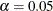is 1.96, which is close to 2. Using this criterion, all estimates shown in Output 29.17.12 are significantly different from zero, supporting the presence of these parameters in the model.

Squared multiple correlations are shown in Output 29.17.13.

Output 29.17.13: Squared Multiple Correlations

Squared Multiple Correlations
Variable Error Variance Total Variance R-Square
Anomie67 3.60796 11.90397 0.6969
Anomie71 3.60796 12.61581 0.7140
Education 2.99366 9.61000 0.6885
Powerless67 3.59488 9.35139 0.6156
Powerless71 3.59488 9.84533 0.6349
SEI 259.57639 450.28798 0.4235
Alien67 5.67046 8.29601 0.3165
Alien71 4.51479 9.00786 0.4988

For each endogenous variable in the model, the corresponding squared multiple correlation is computed by: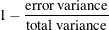In regression analysis, this is the percentage of explained variance of the endogenous variable by the predictors. However, this interpretation is complicated or even uninterpretable when your structural equation model has correlated errors or reciprocal casual relations. In these situations, it is not uncommon to see negative R-squares. Negative R-squares do not necessarily mean that your model is wrong or the model prediction is weak. Rather, the R-square interpretation is questionable in these situations.

When your variables are measured on different scales, comparison of path coefficients cannot be made directly. For example, in Output 29.17.12, the path coefficient for path Education <=== SES is fixed at one, while the path coefficient for path SEI <=== SES is 5.369. It would be simple-minded to conclude that the effect of SES on SEI is greater than that SES on Education. Because SEI and Education are measured on different scales, direct comparison of the corresponding path coefficients is simply inappropriate.

In alleviating this problem, some might resort to the standardized solution for a better comparison. In a standardized solution, because the variances of manifest variables and systematic predictors are all standardized to ones, you hope the path coefficients are more comparable. In this example, PROC CALIS standardizes your results in Output 29.17.14.

Output 29.17.14: Standardized Results

Standardized Results for PATH List
Path Parameter Estimate Standard
Error
t Value Pr > |t|
Anomie67 <=== Alien67   0.83481 0.01093 76.3531 <.0001
Powerless67 <=== Alien67   0.78459 0.01163 67.4776 <.0001
Anomie71 <=== Alien71   0.84499 0.01031 81.9796 <.0001
Powerless71 <=== Alien71   0.79678 0.01107 71.9626 <.0001
Education <=== SES   0.82975 0.03172 26.1599 <.0001
SEI <=== SES lambda 0.65079 0.03019 21.5533 <.0001
Alien67 <=== SES gamma1 -0.56257 0.03456 -16.2796 <.0001
Alien71 <=== SES gamma2 -0.20642 0.04483 -4.6043 <.0001
Alien71 <=== Alien67 beta 0.56920 0.04066 14.0000 <.0001

Standardized Results for Variance Parameters
Variance
Type
Variable Parameter Estimate Standard
Error
t Value Pr > |t|
Error Anomie67 theta1 0.30309 0.01825 16.6031 <.0001
Powerless67 theta2 0.38442 0.01825 21.0695 <.0001
Anomie71 theta1 0.28599 0.01742 16.4178 <.0001
Powerless71 theta2 0.36514 0.01764 20.6942 <.0001
Education theta3 0.31152 0.05264 5.9182 <.0001
SEI theta4 0.57647 0.03930 14.6680 <.0001
Alien67 psi1 0.68352 0.03888 17.5797 <.0001
Alien71 psi2 0.50121 0.03321 15.0897 <.0001
Exogenous SES phi 1.00000

Standardized Results for Covariances Among Errors
Error of Error of Parameter Estimate Standard
Error
t Value Pr > |t|
Anomie67 Anomie71 theta5 0.07391 0.01013 7.2957 <.0001
Powerless67 Powerless71 theta5 0.09440 0.01274 7.4092 <.0001

Now, the standardized path coefficient for path Education <=== SES is 0.830, while the standardized path coefficient for path SEI <=== SES is 0.651. So the standardized effect of SES on SEI is actually smaller than that of SES on Education.

Furthermore, in PROC CALIS the standardized estimates are computed with standard error estimates and t values so that you can make statistical inferences on the standardized estimates as well.

PROC CALIS might differ from other software in its standardization scheme. Unlike other software that might standardize the path coefficients that attach to the error terms (unsystematic sources), PROC CALIS keeps these path coefficients at ones (not shown in the output). Unlike other software that might also standardize the corresponding error variances to ones, the error variances in the standardized solution of PROC CALIS are rescaled so as to keep the mathematical consistency of the model.

Essentially, in PROC CALIS only variances of manifest and non-error-type latent variables are standardized to ones. The error variances are rescaled, but not standardized. For example, in the standardized solution shown in Output 29.17.14, the error variances for all endogenous variables are not ones (see the middle portion of the output). Only the variance for the latent variable SES is standardized to one. See the section Standardized Solutions for the logic of the standardization scheme adopted by PROC CALIS.

In appearance, the standardized solution is like a correlational analysis on the standardized manifest variables with standardized exogenous latent factors. Unfortunately, this statement is over-simplified, if not totally inappropriate. In standardizing a solution, the implicit equality constraints are likely destroyed. In this example, the unstandardized error variances for Anomie67 and Anomie71 are both 3.608, represented by a common parameter theta1. However, after standardization, these error variances have different values at 0.303 and 0.286, respectively. In addition, fixed parameter values are no longer fixed in a standardized solution (for example, the first five paths in the current example). The issue of standardization is common to all other SEM software and beyond the current discussion. PROC CALIS provides the standardized solution so that users can interpret the standardized estimates whenever they find them appropriate.